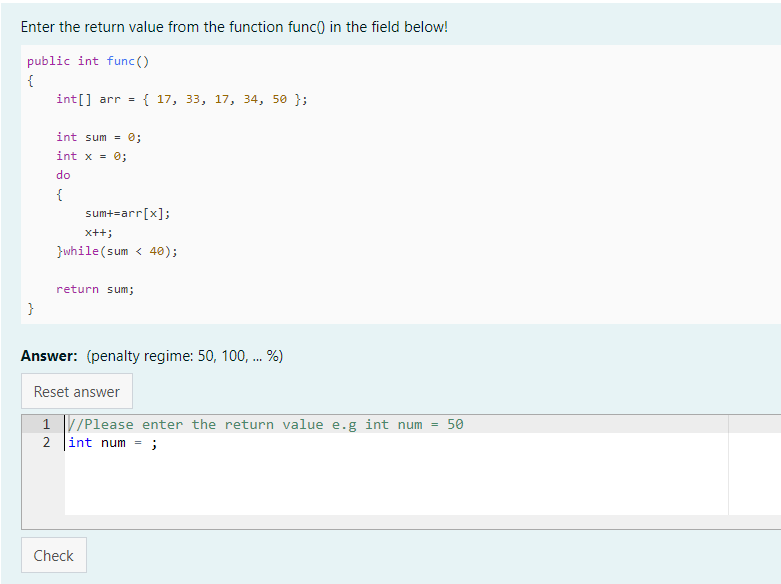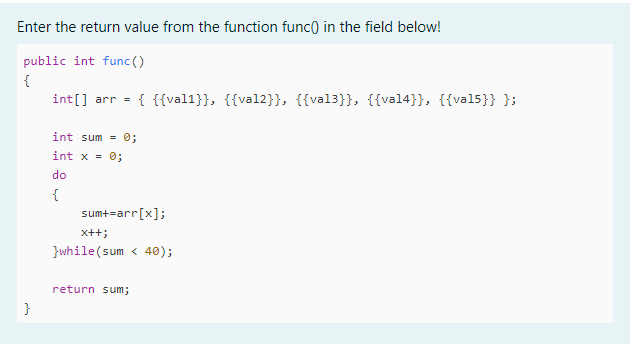by Andreas Pötscher -
Number of replies: 1

I really like code runner and I use it a lot in Classroom with my students.

Now I am writing java Code Reading Questions. Where the Students get a java function and must answer its return value.I use a lot of random variables in this questions, which I create in the Template params section. For testing the correct answer I put the function code in the Global Extra section and use it afterwards the tester class. In the testcases the student answer is compared with the return value from the function. The student answer is also tested for some forbidden keywords e.g func().

This type of question works very well, so I decided to write a question template. With this some questions occured.

• I would like to use the code from the Global extra section in the Question Text. Which can be done with {{ QUESTION.globalextra }}. But the problem here is. If I use random template params, the Question text is processed first and the template params in the global extra are not replaced before the global extra is replaced in the Question text. Is there a way to change the order of processing? The advantage would be to write the function only once. Using Globalextra looks like this:• For making more questions of this type the test cases are allways the same. Is it possible to put the testcases in the question template?

For better understanding I attatched a demo question.

Greetings Andi### Re: Java Code Reading Question

by Richard Lobb -

Hi Andreas

That's an interesting example.

I'm afraid that changing the order of Twig evaluation of the various fields of the question wouldn't help. When the question is first initialised, the template parameter field is evaluated by Twig (in your case). The result is a JSON record that provides a set of variables which then provide the environment for all subsequent Twig evaluations, namely the question text, each test case etc and ultimately, when the question is submitted, the template itself. However, all those evaluations are independent - there is no state preserved across the different evaluations. So changing the order in which fields of the question are evaluated wouldn't help. It's just not possible to have the global extra expanded by Twig and then to use that expanded version in a subsequent field of the question.

To make your approach work you would need to define the function func in the template parameter field. But the template parameters are JSON, and JSON doesn't allow proper multiline strings, which would make any attempt to define your multiline func very clumsy and unmaintainable. Which is, I assume, the reason you put it into the global extra instead.

BUT ... there is a solution! The latest version of CodeRunner (4.0.1) allows you to use languages other than Twig for constructing randomised template parameters. See here. Python would be my choice. If you select python3 as the template parameter preprocessor you can write your template parameters as

import sys, json
from random import seed, randint

args = {param.split('='): param.split('=') for param in sys.argv[1:]}
seed(args['seed'])

operators = ["<", "<=", ">", ">=", "==", "!="]
val1 = randint(0, 4)
val2 = randint(0, 4)
val3 = randint(10, 50)
val4 = randint(10, 50)
val5 = randint(10, 50)
var = operators[randint(0, len(operators)) - 1]  # Unused?

func = f"""public int func()
{{
int[] arr = {{ {val1}, {val2}, {val3}, {val4}, {val5} }};

int sum = 0;
int x = 0;
do
{{
sum += arr[x];
x++;
}} while (sum < 40);

return sum;
}}
"""

print(json.dumps({"func": func}))

The output of that Python program is a JSON template parameter string that just defines the single value func. It is a little clumsy that the Java braces all have to be doubled to prevent their being interpreted as Python f-string format characters, but is otherwise workable enough?

With that approach, your question text is now just

Enter the return value from the function func() in the field below!


{{ func }}


This new preprocessor capability is still somewhat experimental but we've used it a bit in a course with over 1000 students and it worked perfectly. I attach an xml export of your question written that way.

You'll see that there are several warnings about using non-Twig preprocessors in tests and exams. However, if you're using Java questions, I think you're far more likely to overload the Jobe server with actual Java submissions in a test than by using a Python preprocessor. I would suggest, though, that using Java as the preprocessor (which is also possible) would be asking for trouble in tests and exams.